Question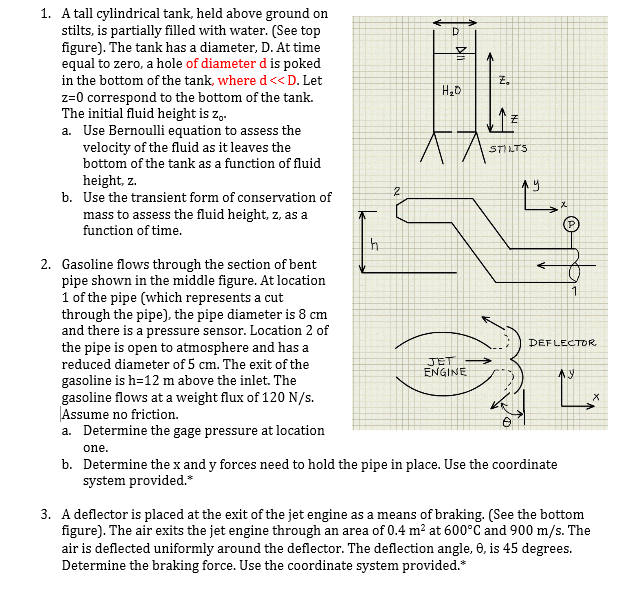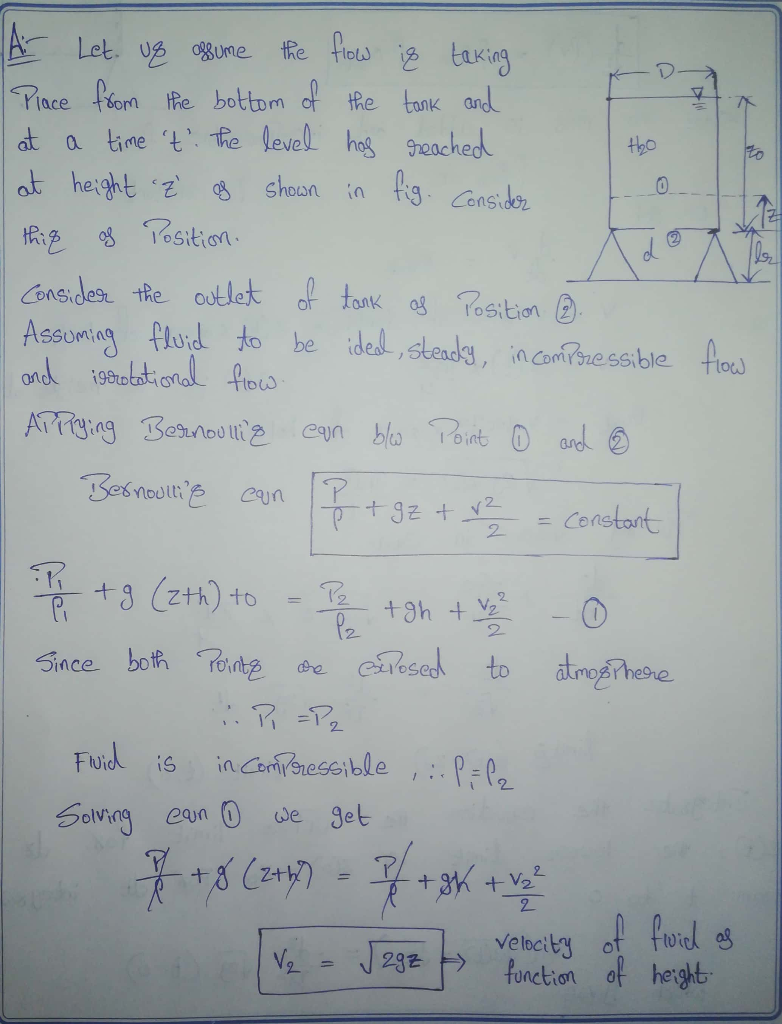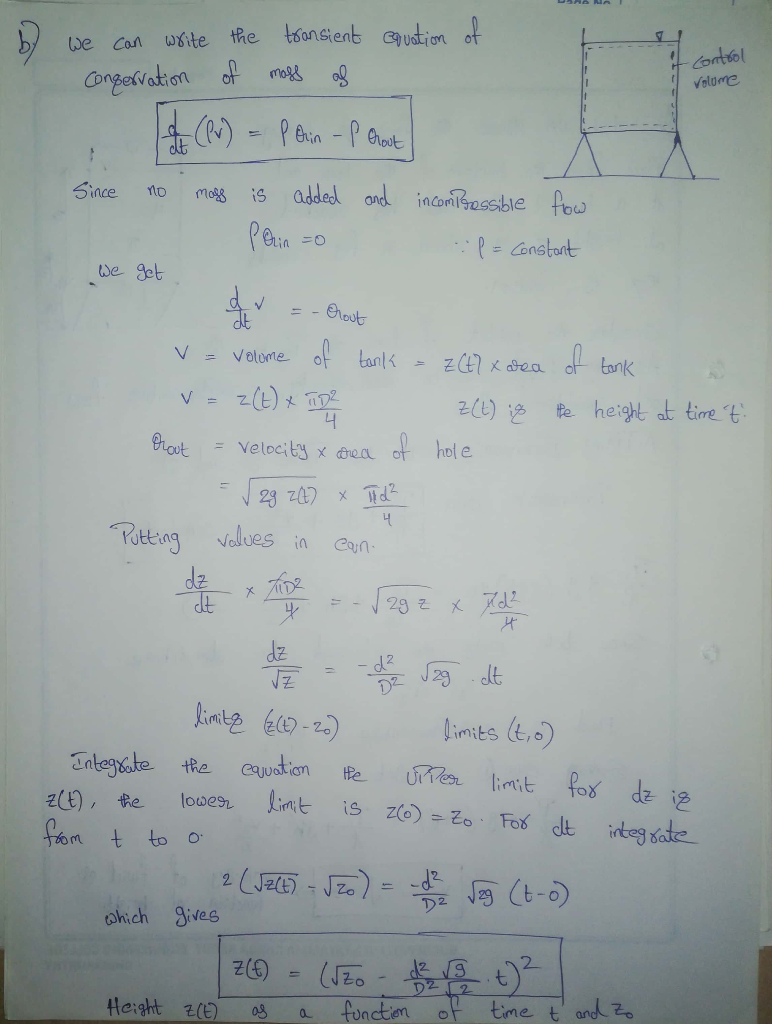#### Earn Coins

Coins can be redeemed for fabulous gifts.

Similar Homework Help Questions
• ### Water flows steadily from a large tank as shown in the figure. The height of water...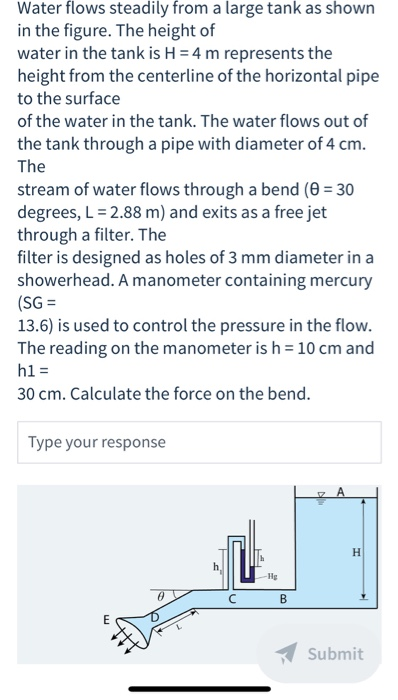Water flows steadily from a large tank as shown in the figure. The height of water in the tank is H = 4 m represents the height from the centerline of the horizontal pipe to the surface of the water in the tank. The water flows out of the tank through a pipe with diameter of 4 cm. The stream of water flows through a bend (= 30 degrees, L = 2.88 m) and exits as a free jet through...

• ### The figure shows a cylindrical tank of 80 em in diameter which is fully filled with water. In order to increase the flow from the tank to the exit pipe on the left, an additional pressure is appl...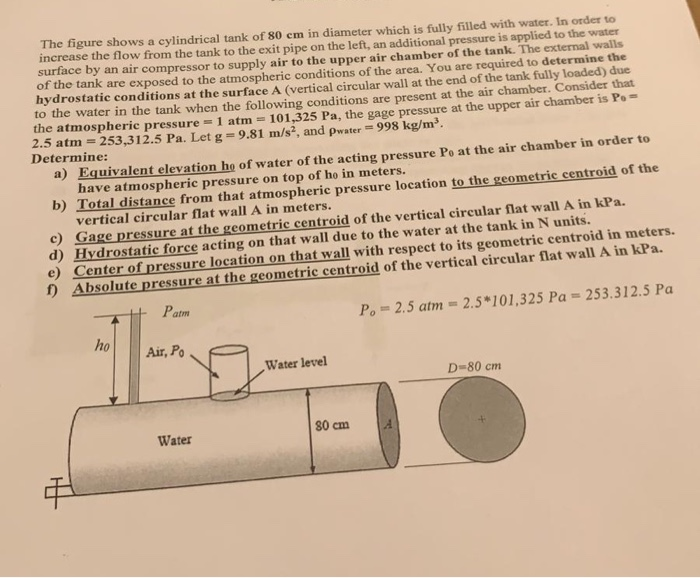The figure shows a cylindrical tank of 80 em in diameter which is fully filled with water. In order to increase the flow from the tank to the exit pipe on the left, an additional pressure is applied to the water surface by an air compressor to supply air to the upper air chamber of the tank. The external walls of the tank are exposed to the atmospheric conditions of the area. You are required to determine the hydrostatic conditions...

• ### 8. (15 points) Water flows through a 3-in diameter pipe with a velocity of 25 ft/s...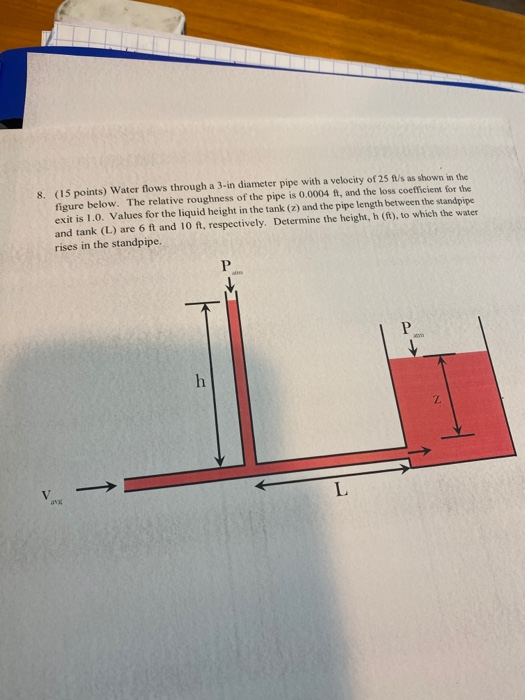8. (15 points) Water flows through a 3-in diameter pipe with a velocity of 25 ft/s as shown in the figure below. The relative roughness of the pipe is 0.0004 ft, and the loss coefficient for the exit is 1.0. Values for the liquid height in the tank (z) and the pipe length between the standpipe and tank (L) are 6 ft and 10 ft, respectively. Determine the height, h (ft), to which the water rises in the standpipe. P...

• ### A fluid of density 1300 kg/m3 flows out through a 3 meter diameter pipe without friction at a rat...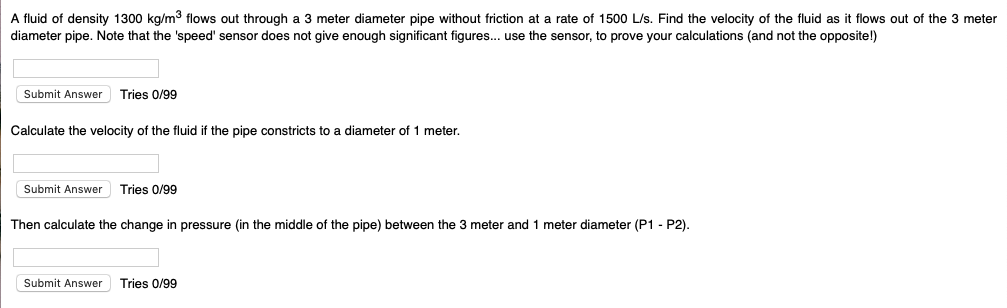A fluid of density 1300 kg/m3 flows out through a 3 meter diameter pipe without friction at a rate of 1500 L/s. Find the velocity of the fluid as it flows out of the 3 meter diameter pipe. Note that the 'speed' sensor does not give enough significant figures... use the sensor, to prove your calculations (and not the opposite!) Submit Answer Tries 0/99 Calculate the velocity of the fluid if the pipe constricts to a diameter of 1 meter....

• ### A 3-m-diameter tank is initially filled with water 2 m above the center of a sharp-edged 10-cm-diameter orifice. The tank water surface is open to the atmosphere, and the orifice drains to the at...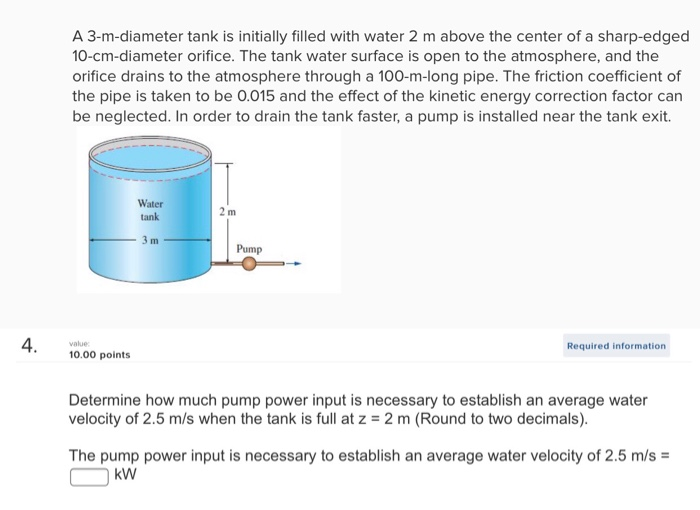A 3-m-diameter tank is initially filled with water 2 m above the center of a sharp-edged 10-cm-diameter orifice. The tank water surface is open to the atmosphere, and the orifice drains to the atmosphere through a 100-m-long pipe. The friction coefficient of the pipe is taken to be 0.015 and the effect of the kinetic energy correction factor can be neglected. In order to drain the tank faster, a pump is installed near the tank exit. Water tank Pump Required...

• ### (3 points) A tank of diameter D is filled with water up to a height h...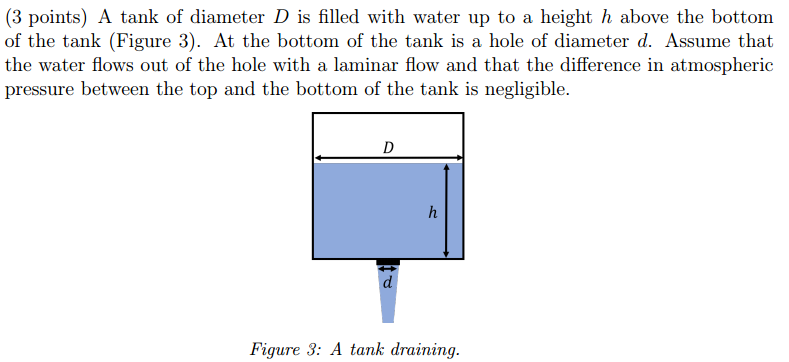(3 points) A tank of diameter D is filled with water up to a height h above the bottom of the tank (Figure 3). At the bottom of the tank is a hole of diameter d. Assume that the water flows out of the hole with a laminar flow and that the difference in atmospheric pressure between the top and the bottom of the tank is negligible. Figure 3: A tank draining (c) If you no longer assume that the...

• ### Problem 1. Water flows from a large tank through a smooth pipe of length 80 m....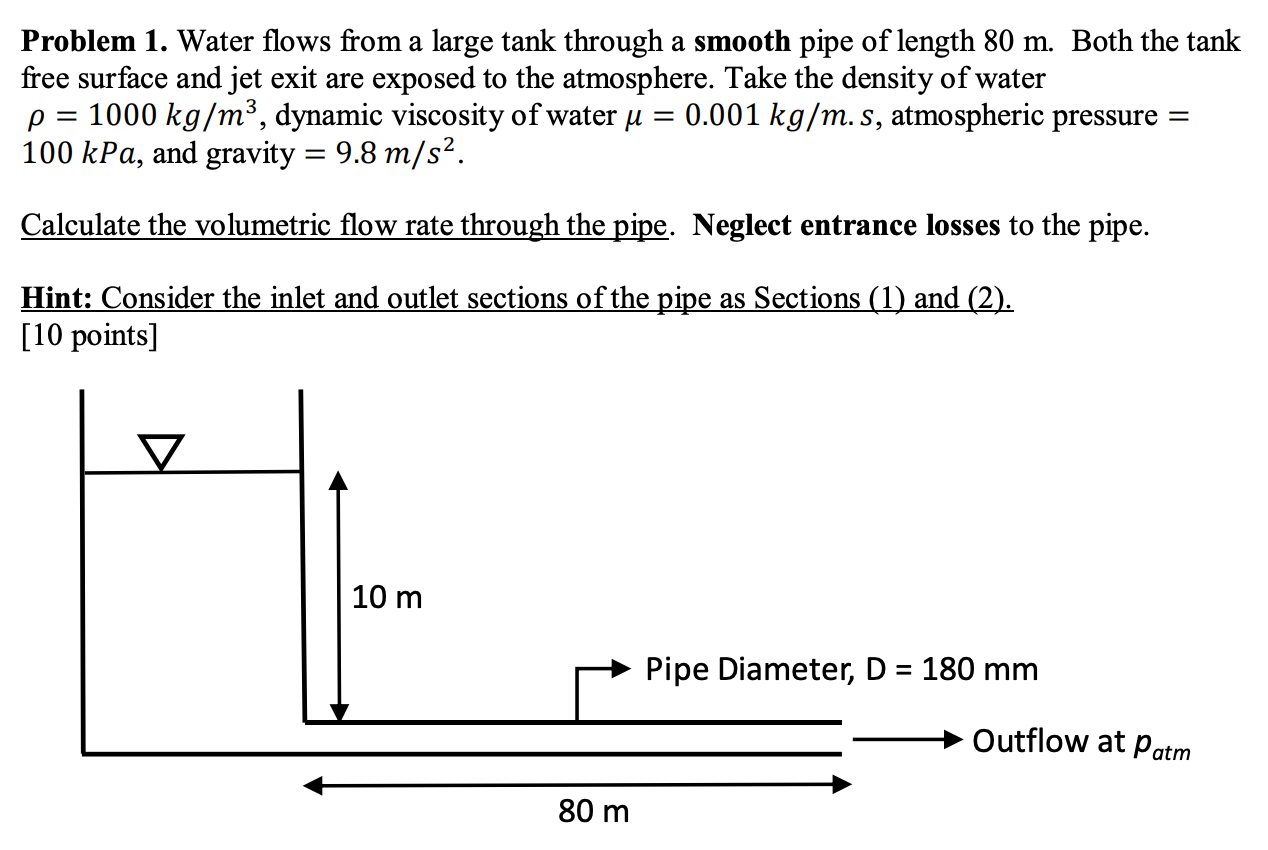Problem 1. Water flows from a large tank through a smooth pipe of length 80 m. Both the tank free surface and jet exit are exposed to the atmosphere. Take the density of water p = 1000 kg/m3, dynamic viscosity of water u = 0.001 kg/m.s, atmospheric pressure = 100 kPa, and gravity = 9.8 m/s2. Calculate the volumetric flow rate through the pipe. Neglect entrance losses to the pipe. Hint: Consider the inlet and outlet sections of the pipe...

• ### 8. (15 points) Water flows through a 2-in diameter pipe with a velocity of 15 ft/s...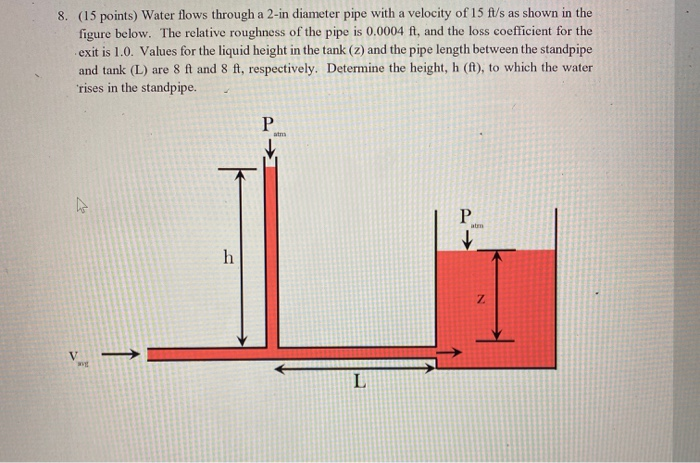8. (15 points) Water flows through a 2-in diameter pipe with a velocity of 15 ft/s as shown in the figure below. The relative roughness of the pipe is 0.0004 ft, and the loss coefficient for the exit is 1.0. Values for the liquid height in the tank (2) and the pipe length between the standpipe and tank (L) are 8 ft and 8 ft, respectively. Determine the height, h (ft), to which the water rises in the standpipe. P...

• ### A 3-m-diameter tank is initially filled with water 2 m above the center of a sharp-edg...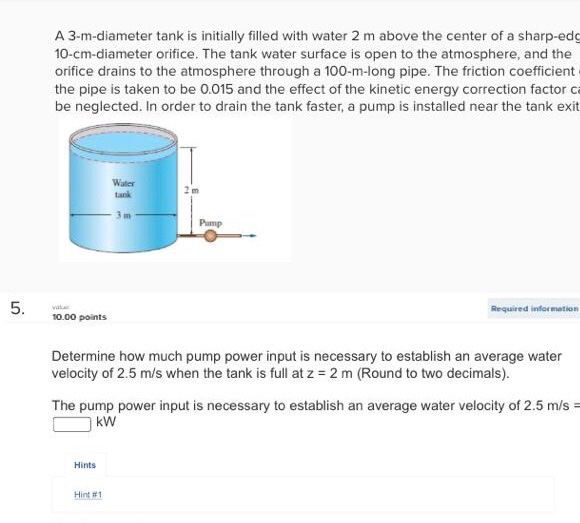A 3-m-diameter tank is initially filled with water 2 m above the center of a sharp-edg 10-cm-diameter orifice. The tank water surface is open to the atmosphere, and the orifice drains to the atmosphere through a 100-m-long pipe. The friction coefficient the pipe is taken to be 0.015 and the effect of the kinetic energy correction factor ca be neglected. In order to drain the tank faster, a pump is installed near the tank exit Water tank Pamp Required infor...

• ### Problem 1. Water flows from a large tank through a smooth pipe of length 80 m....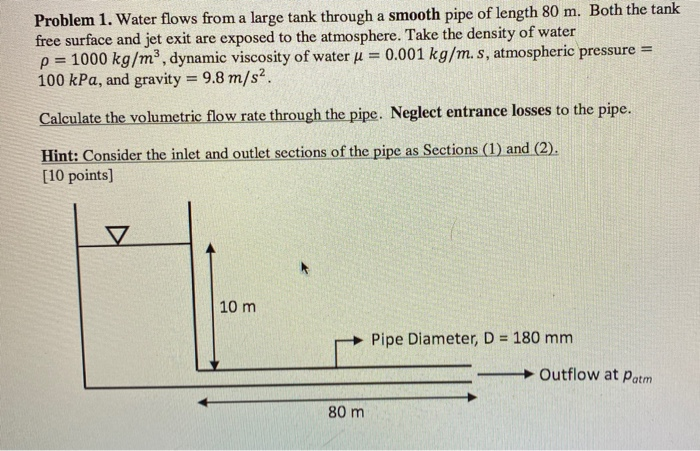Problem 1. Water flows from a large tank through a smooth pipe of length 80 m. Both the tank free surface and jet exit are exposed to the atmosphere. Take the density of water p = 1000 kg/m3, dynamic viscosity of water j = 0.001 kg/m.s, atmospheric pressure = 100 kPa, and gravity = 9.8 m/s2. Calculate the volumetric flow rate through the pipe. Neglect entrance losses to the pipe. Hint: Consider the inlet and outlet sections of the pipe...